# 二叉排序树

``````左子树节点<根节点值<右子树节点，对于二叉排序树进行中序遍历，可以得到一个递增序列,下图中序遍历序列为:1 2 3 4 6 8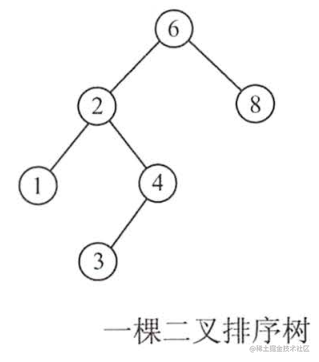``````1)若删除节点z是叶节点，则直接删除
2)若z只有一颗左子树或右子树，则让z的子树代替z的位置(用子女代替)
3)若z有左右子树，找第一个子女来填补

2图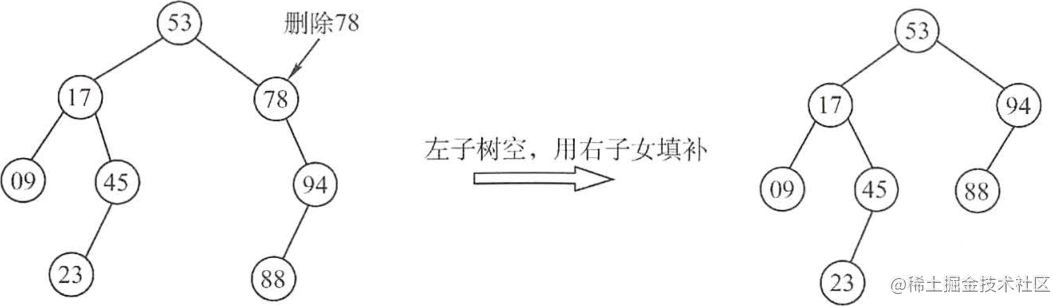3图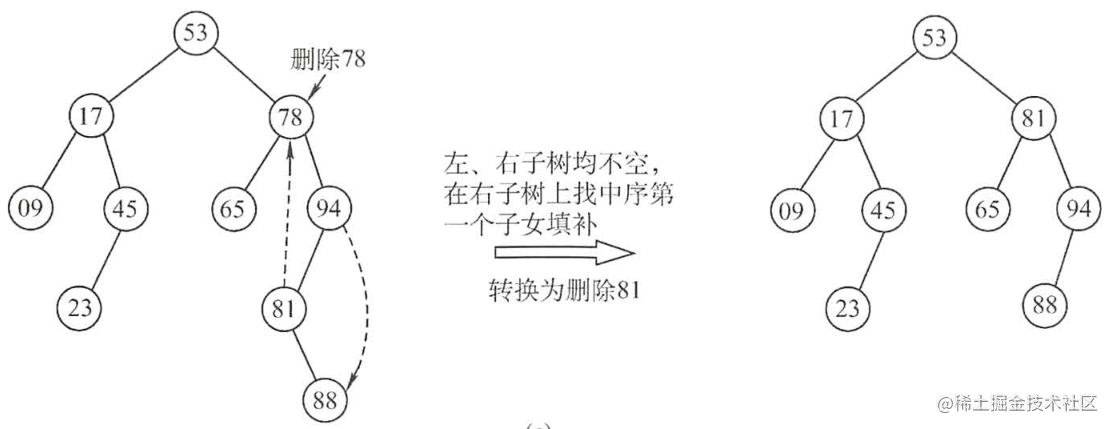``````上图的查找效率为 ASL=(1+2*2+3*4+4*3)/10=2.9

## 平衡二叉树

``````任意节点的`左右子树高度差的绝对值不超过1`，这样的二叉树称为'平衡二叉树';

• -->平衡 如下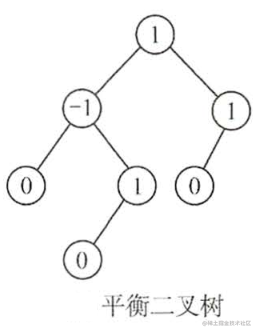• -->不平衡 如下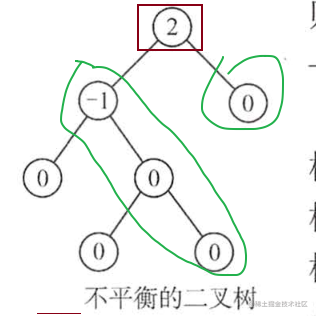## ※平衡二叉树的插入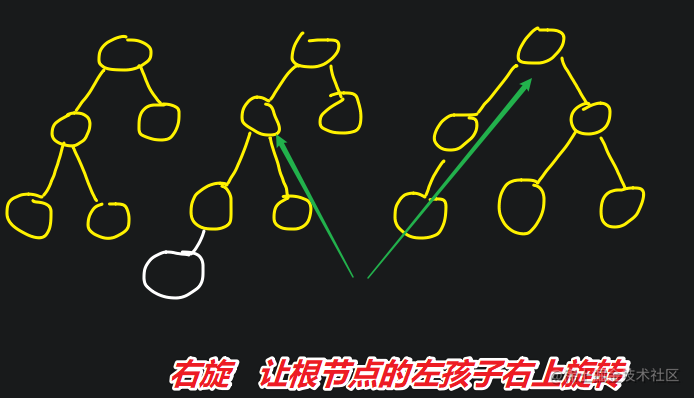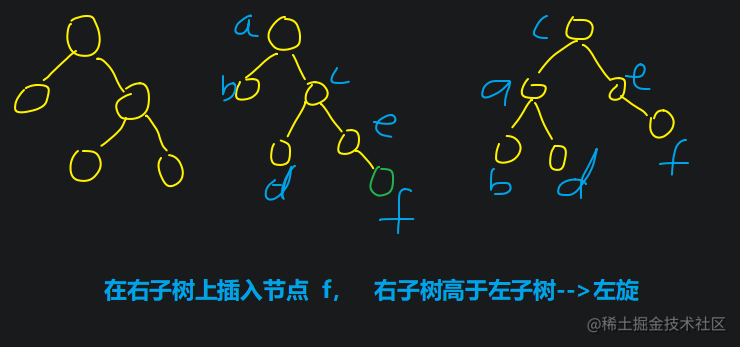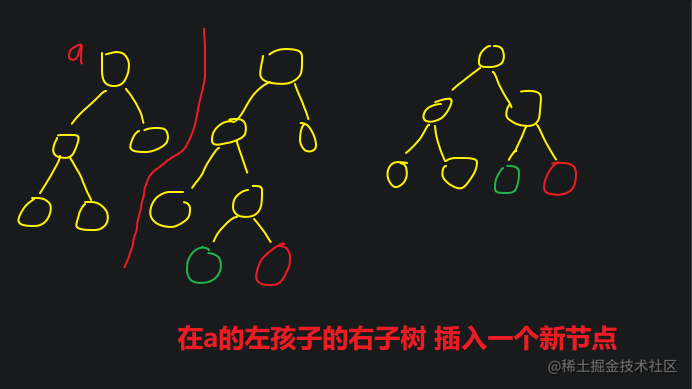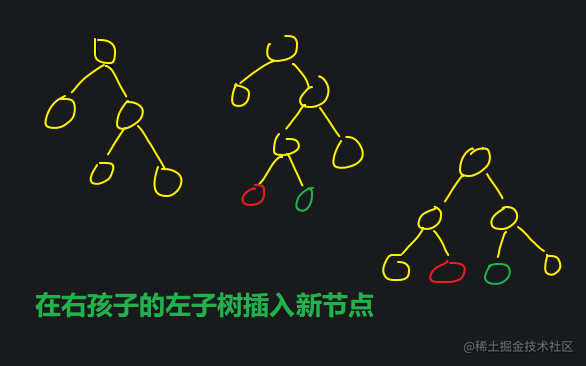## 哈夫曼树

• (a) WPL=7×2+5×2+2×2+4×2=36
• (b) WPL = 7×3+5×3+4×2+1×2=46
• (c)WPL=7×1+5×2+2×3+4×3=35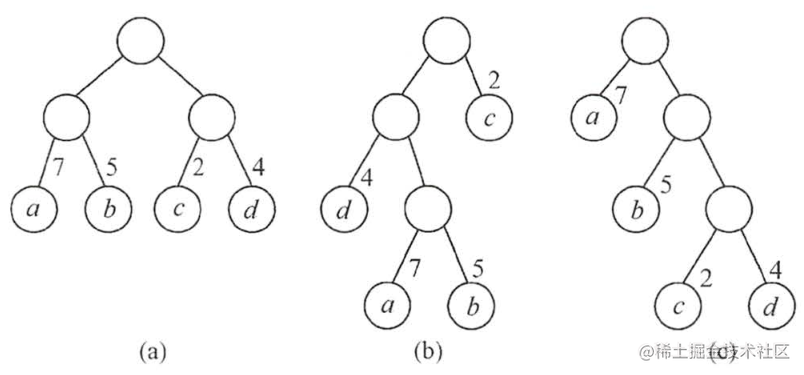## 哈夫曼树的构造

• 森林中选择出两颗结点的'权值最小'的二叉树
• 合并两颗二叉树，增加一个新的节点作为'新的二叉树的根'，权值为'左右孩子的权值之和'